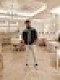Question Paper: Production Planning And Control Question Paper - Dec 17 - Mechanical Engineering (Semester 7) - Mumbai University (MU)
0

Production Planning And Control - Dec 17

Mechanical Engineering (Semester 7)

Total marks: 80
Total time: 3 Hours
INSTRUCTIONS
(1) Question 1 is compulsory.
(2) Attempt any three from the remaining questions.
(3) Draw neat diagrams wherever necessary.

1 Answer any four

1.a. What are the components and types for a manufacturing system.
(5 marks) 00

1.b. What are pre requisites of PPC.
(5 marks) 00

1.c. Define the terms : lead time, safety stock, reorder point and maximum inventory.
(5 marks) 00

1.d. What are the characteristics of forecasting?
(5 marks) 00

1.e. Explain optimistic time, most likely time and pessimistic time for PERT.
(5 marks) 00

2.a. How the size of an organization affects the various factors which influence the PPC
(10 marks) 00

2.b. What is a work order? What is its importance? Explain with suitable example.
(10 marks) 00

3.a. The demand for an item is deterministic and constant over time and is equal to 600 units per year. The unit cost of the item is Rs. 50 while the cost of placing an order is Rs. 5. The inventory carrying cost is 20% of the cost of the inventory per annum and the cost of shortage is Rs. 1 per month. Find the optimal ordering quantity when stock outs are permitted. If the stock outs are not permitted what would be the loss to the company.
(10 marks) 00

3.b. Explain any one with example:

i. Two bin system of inventory control

ii. ABC analysis for inventory control

(10 marks) 00

4.a. Why process planning is needed? Explain the various types of Computer Aided Process Planning
(10 marks) 00

4.b. The following data gives the sales of the company for the various years. Fit the straight line and forecast the sales for the year 2018 and 2019. [ Tabulate the calculations]

Year 2009 2010 2011 2012 2013 2014 2015 2016 2017
Sale Rs.(000) 13 20 20 28 30 32 33 38 43

(10 marks) 00

5.a. Discuss the importance of process planning. Also discuss in brief the types of process planning
(10 marks) 00

5.b. A company has three factories X, Y , Z . It supplies goods to four warehouses W1,W2,W3 and W4. The production capacities of the factories and the demand of the warehouses are as shown in the table. Determine the optimal solution of the problem.(10 marks) 00

6.a. Consider the LPP and solve by simplex method

Maximize $Z=4 X_{1}+3 X_{2}+6 X_{3}$

Subject to

$2 X_{1}+3 X_{2}+2 X_{3} \leq 440$

$4 X_{1}+32 X_{3} \leq 470$

$2 X_{1}+5 X_{2} \leq 430$

$\mathrm{X}_{1}, \mathrm{X}_{2}, \mathrm{X}_{3} \geq 0$

(10 marks) 00

6.b. A Project consist of following six activities :

Activity Normal time N(t) Crash time (ct) Normal cost Crash cost
1-2 3 2 1600 1800
1-3 7 5 1400 2000
2-3 5 3 2500 3000
3-4 4 3 500 800
3-5 2 1 4200 4400
4-5 8 6 1600 2600

i. Draw the network for the activities stated above

ii. Identify the critical path

iii. What is Total Project duration and associated cost.

iv. If the duration of project t be reduced by 1 week, which activity or activities duration to be reduced? What will be the total project cost?

(10 marks) 00

 modified 4 months ago  • written 4 months ago byneelchheda45 • 0